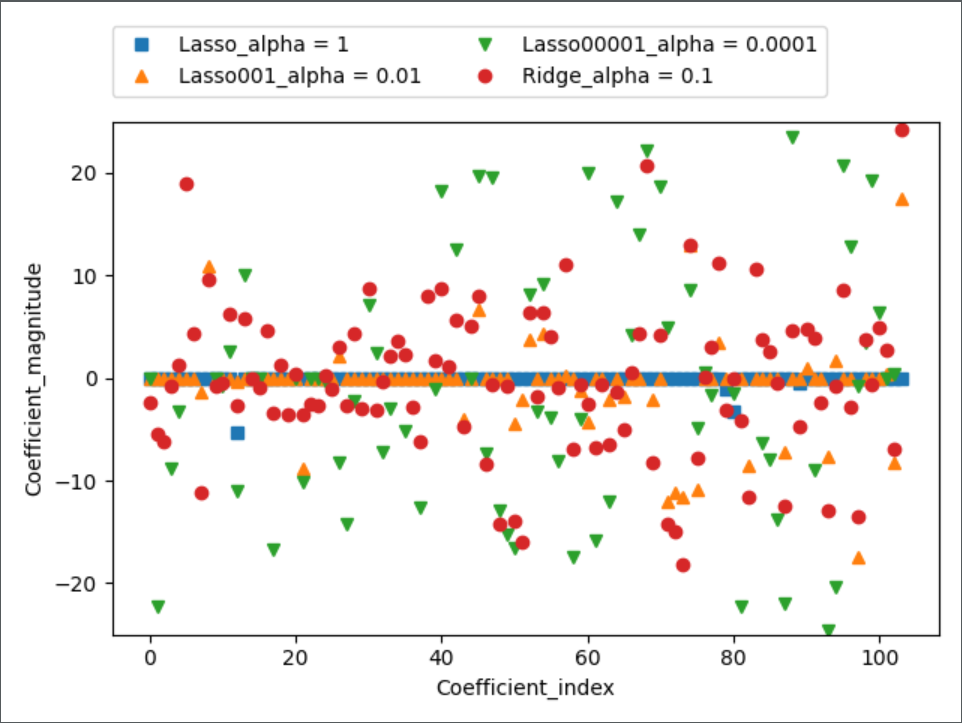# lasso

The Lasso 是估计稀疏系数的线性模型。 它在一些情况下是有用的，因为它倾向于使用具有较少参数值的情况，有效地减少给定解决方案所依赖变量的数量。 因此，Lasso 及其变体是压缩感知领域的基础。 在一定条件下，它可以恢复一组非零权重的精确集。

m i n   w 1 2 n s a m p l e s ∣ ∣ X w − y ∣ ∣ 2 2 + α ∣ ∣ w ∣ ∣ 1 \underset{w}{min\,} { \frac{1}{2n_{samples}} ||X w - y||_2 ^ 2 + \alpha ||w||_1}
lasso estimate 解决了加上罚项 α ∣ ∣ w ∣ ∣ 1 \alpha ||w||_1 的最小二乘法的最小化，其中， α \alpha 是一个常数， ∣ ∣ w ∣ ∣ 1 ||w||_1 是参数向量的 ℓ 1 − n o r m \ell_1-norm 范数。
Lasso 类的实现使用了 coordinate descent （坐标下降算法）来拟合系数。

>>> from sklearn import linear_model
>>> reg = linear_model.Lasso(alpha = 0.1)
>>> reg.fit([[0, 0], [1, 1]], [0, 1])
Lasso(alpha=0.1, copy_X=True, fit_intercept=True, max_iter=1000,
normalize=False, positive=False, precompute=False, random_state=None,
selection='cyclic', tol=0.0001, warm_start=False)
>>> reg.predict([[1, 1]])
array([ 0.8])


alpha 参数控制估计系数的稀疏度。

scikit-learn 通过交叉验证来公开设置 Lasso alpha 参数的对象:LassoCVLassoLarsCVLassoLarsCV是基于下面解释的 最小角回归 算法。

alpha 和 SVM 的正则化参数C 之间的等式关系是 alpha = 1 / C 或者 alpha = 1 / (n_samples * C) ，并依赖于估计器和模型优化的确切的目标函数。

L1正则化的结果是，使用lasso时某些系数刚好为0。这说明某些特征被模型完全忽略。这可以看作是一种自动化的特征选择。

lasso = Lasso().fit(X_train, y_train)

# 训练集和测试集的性能
print("Training set score: {:.2f}".format(lasso.score(X_train, y_train)))
print("Test set score: {:.2f}".format(lasso.score(X_test, y_test)))
print("Number of feature used: {}".format(np.sum(lasso.coef_ != 0)))

=================================

Training set score: 0.29
Test set score: 0.21
Number of feature used: 4


lasso001 = Lasso(alpha=0.01, max_iter=100000).fit(X_train, y_train)

# 训练集和测试集的性能
print("Training set score: {:.2f}".format(lasso001.score(X_train, y_train)))
print("Test set score: {:.2f}".format(lasso001.score(X_test, y_test)))
print("Number of feature used: {}".format(np.sum(lasso001.coef_ != 0)))

===================================
Training set score: 0.90
Test set score: 0.77
Number of feature used: 33


alpha值变小，我们可以拟合一个更复杂的模型，在训练集和测试集上的表现也更好。模型性能比使用Ridge时略好一点，而且我们只用到了105个特征中的33个。这样模型可能更容易理解。

lasso00001 = Lasso(alpha=0.0001, max_iter=100000).fit(X_train, y_train)

# 训练集和测试集的性能
print("Training set score: {:.2f}".format(lasso00001.score(X_train, y_train)))
print("Test set score: {:.2f}".format(lasso00001.score(X_test, y_test)))
print("Number of feature used: {}".format(np.sum(lasso00001.coef_ != 0)))

====================================
Training set score: 0.95
Test set score: 0.64
Number of feature used: 96


plt.plot(lasso.coef_, 's', label="Lasso_alpha = 1")
plt.plot(lasso001.coef_, '^', label="Lasso001_alpha = 0.01")
plt.plot(lasso00001.coef_, 'v', label="Lasso00001_alpha = 0.0001")
plt.plot(ridge01.coef_, 'o', label="Ridge_alpha = 0.1")

plt.xlabel("Coefficient_index")
plt.ylabel("Coefficient_magnitude")
plt.ylim(-25, 25)
plt.legend(ncol=2, loc=(0, 1.05))

plt.show()# 完整代码

from sklearn.model_selection import train_test_split
from sklearn.linear_model import LinearRegression
from sklearn.linear_model import Ridge
from sklearn.linear_model import Lasso

import numpy as np
import mglearn as mglearn
import matplotlib.pyplot as plt

X_train, X_test, y_train, y_test = train_test_split(X, y, random_state=0)

##################### LinearRegression

lr = LinearRegression().fit(X_train, y_train)

# 训练集和测试集的性能
print("\n[LinearRegression]")
print("Training set score: {:.2f}".format(lr.score(X_train, y_train)))
print("Test set score: {:.2f}".format(lr.score(X_test, y_test)))

##################### Ridge

########### Ridge_alpha = 1

ridge = Ridge().fit(X_train, y_train)

# 训练集和测试集的性能
print("\n[Ridge_alpha = 1]")
print("Training set score: {:.2f}".format(ridge.score(X_train, y_train)))
print("Test set score: {:.2f}".format(ridge.score(X_test, y_test)))

########## Ridge_alpha = 10

ridge10 = Ridge(alpha=10).fit(X_train, y_train)

# 训练集和测试集的性能
print("\n[Ridge_alpha = 10]")
print("Training set score: {:.2f}".format(ridge10.score(X_train, y_train)))
print("Test set score: {:.2f}".format(ridge10.score(X_test, y_test)))

########## Ridge_alpha = 0.1

ridge01 = Ridge(alpha=0.1).fit(X_train, y_train)

# 训练集和测试集的性能
print("\n[Ridge_alpha = 0.1]")
print("Training set score: {:.2f}".format(ridge01.score(X_train, y_train)))
print("Test set score: {:.2f}".format(ridge01.score(X_test, y_test)))

#################### Lasso

########## Lasso_alpha = 1

lasso = Lasso().fit(X_train, y_train)

# 训练集和测试集的性能
print("\n[Lasso_alpha = 1]")
print("Training set score: {:.2f}".format(lasso.score(X_train, y_train)))
print("Test set score: {:.2f}".format(lasso.score(X_test, y_test)))
print("Number of feature used: {}".format(np.sum(lasso.coef_ != 0)))

########## Lasso_alpha = 0.01

lasso001 = Lasso(alpha=0.01, max_iter=100000).fit(X_train, y_train)

# 训练集和测试集的性能
print("\n[Lasso_alpha = 0.01]")
print("Training set score: {:.2f}".format(lasso001.score(X_train, y_train)))
print("Test set score: {:.2f}".format(lasso001.score(X_test, y_test)))
print("Number of feature used: {}".format(np.sum(lasso001.coef_ != 0)))

########## Lasso_alpha = 0.0001

lasso00001 = Lasso(alpha=0.0001, max_iter=100000).fit(X_train, y_train)

# 训练集和测试集的性能
print("\n[Lasso_alpha = 0.0001]")
print("Training set score: {:.2f}".format(lasso00001.score(X_train, y_train)))
print("Test set score: {:.2f}".format(lasso00001.score(X_test, y_test)))
print("Number of feature used: {}".format(np.sum(lasso00001.coef_ != 0)))

# #################### 制图
# plt.plot(ridge.coef_, 's', label="Ridge_alpha = 1")
# plt.plot(ridge10.coef_, '^', label="Ridge_alpha = 10")
# plt.plot(ridge01.coef_, 'v', label="Ridge_alpha = 0.1")
# plt.plot(lr.coef_, 'o', label="LinearRegression")
#
# plt.xlabel("Coefficient_index")
# plt.ylabel("Coefficient_magnitude")
# plt.hlines(0, 0, len(lr.coef_))
# plt.ylim(-25, 25)
# plt.legend()
#
# mglearn.plots.plot_ridge_n_samples()
# plt.show()

plt.plot(lasso.coef_, 's', label="Lasso_alpha = 1")
plt.plot(lasso001.coef_, '^', label="Lasso001_alpha = 0.01")
plt.plot(lasso00001.coef_, 'v', label="Lasso00001_alpha = 0.0001")
plt.plot(ridge01.coef_, 'o', label="Ridge_alpha = 0.1")

plt.xlabel("Coefficient_index")
plt.ylabel("Coefficient_magnitude")
plt.ylim(-25, 25)
plt.legend(ncol=2, loc=(0, 1.05))

plt.show()



### Hadoop HA --- 网络原因/NN Full GC造成集群故障_Miracle_Sylvain的博客-程序员宅基地

Hadoop HA — 网络原因/NN Full GC造成集群故障1 问题描述简单描述一下问题，集群为Hadoop HDFS HA模式，三个节点104、105、106，由于三个节点的时间不同，以105时间为标准。2017-07-08 01:45分左右，集群出现故障。2 日志信息105 NN 日志如下： 105 DN日志如下： 104 JN日志如下： 105 JN日志如下： 106 JN日志如

### sql access mysql数据库_MySQL_mysql数据复制到access数据库，mysql数据库表sqltable 字段id，nam - phpStudy..._Chevy Shan的博客-程序员宅基地

mysql数据复制到access数据库mysql数据库表sqltable字段id，name，sex，emailaccess数据库表accesstableid，name，sex，email$connect = mysql_connect("localhost","","");mysql_select_db("mydatabase");$sql = "select * from sqltable;\$r...

### Spark 性能优化和故障处理_马本不想再等了的博客-程序员宅基地

Spark 性能优化和故障处理一、Spark 性能优化1.1 常规性能优化生产环境 Spark submit 脚本/usr/local/spark/bin/spark-submit \--class com.atguigu.spark.WordCount \--num-executors 80 \--driver-memory 6g \--executor-memory 6g \...

### Windows IIS 集成PHP时修改PHP.ini 配置后不生效问题_aohuofeng9952的博客-程序员宅基地

iis下修改c://windows/php.ini 重启iis的网站不生效。需要重启应用程序池即可生效。转载于:https://www.cnblogs.com/SXLBlog/p/10406859.html

### java图片透明浮水印_PHP 为图片加上浮水印 (含浮水印透明背景处理)_weixin_39947396的博客-程序员宅基地

PHP 要为图片加上浮水印: 就是要将两张图合并在一起, 浮水印的图片要盖在上面.现实状况还会遇到原图要缩略图后, 浮水印的图片可能也要跟着缩略图, 这些部分再此就不写了, 此部份缩略图可见此篇:此篇只写如何用 PHP GD 来合并图片, 做浮水印功能.PHP 为图片加上浮水印 (含浮水印透明背景处理)PHP 要为图片加上浮水印 (两张图合并), 可用下述 Function:imagecopyme...